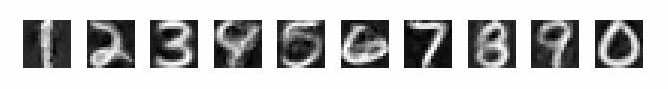Examples: Statistical Pattern Recognition Toolbox Home

The following list contains selected demos and examples implemented in the toolbox:
• Interactive demo on algorithm learning the linear classifiers.
• Interactive demo on algorithm solving the Generalized Anderson's Task.
• Interactive demo on Support Vector Machines.
• Interactive demo on Expectation-Maximization algorithm.
• Interactive demo on Minimax estimation of Gaussian density.
• Example: Training multi-class linear classifier by the Perceptron.
• Example: Principal Component Analysis.
• Example: Comparison between LDA and PCA.
• Example: Greedy Kernel Principal Component Analysis.
• Example: Quadratic classifier trained the Perceptron.
• Example: Probabilistic output for Support Vector Machines.
• Example: K-means clustering.
• Example: Multi-class BSVM with L2-soft margin.
• Example: Kernel Fisher Discriminant.
• Example: Reduced set method for SVM classifier.
• Example: Bayesian classifier with reject option.
• Example: K-nearest neighbors classifier.
• Demo: Optical Character Recognition.
• Demo: Image denoising by the kernel PCA.
• ### Demo: Algorithms learning linear classifiers.

 This demo shows algorithms learning separating hyperplane for binary separable data, e.g., Perceptron, Kozinec's algorithm, linear SVM. The demo allows to create interactively a simple examples and to compare different algorithms.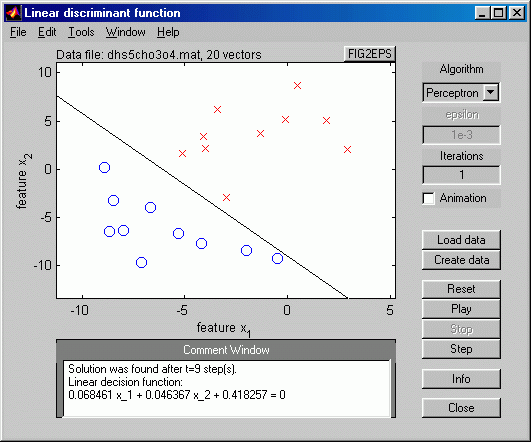### Demo: Algorithms solving the Generalized Anderson's task.

 The Generalized Anderson's task belongs to a class of non-Bayesian approaches for classification. The class-conditional probabilities are assumed to be influenced by a non-random intervention. The minimax approach is used to design a classifier prepared for the worst possible intervention. The demo allows to create interactively a simple examples and to compare different algorithms to solve the task.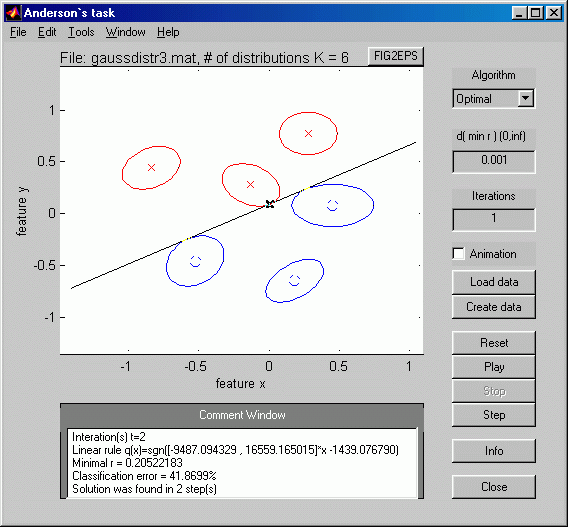### Demo: Support Vector Machines.

 The demo allows to interactively define a toy training sets and to train the SVM classifier with different kernels and regularization constants.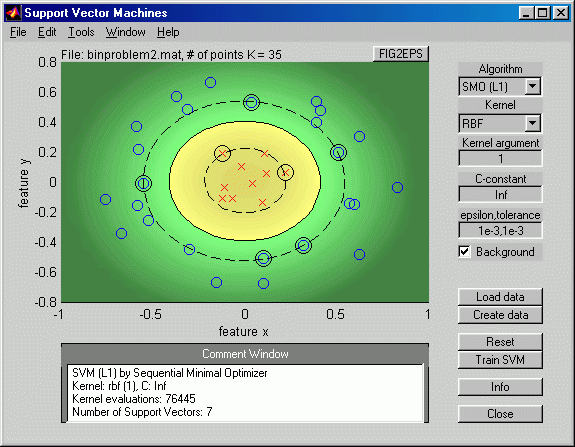### Demo: Expectation-Maximization algorithm.

 The demo shows the EM algorithm used for estimation of parameters of the Gaussian mixture model.### Demo: Minimax estimation of Gaussian parameters.

 The demo shows the minimax algorithm to estimate parameters of multivariate Gaussian distribution.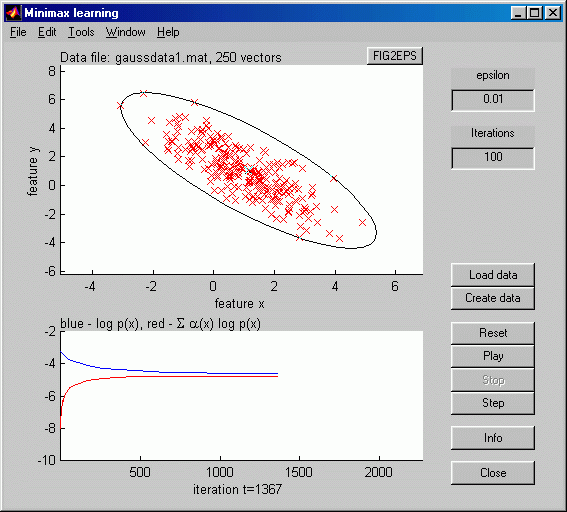### Example: Training multi-class linear classifier by the Perceptron.

 The example shows application of the Perceptron rule to train the multi-class linear classifier using the Kesler's construction.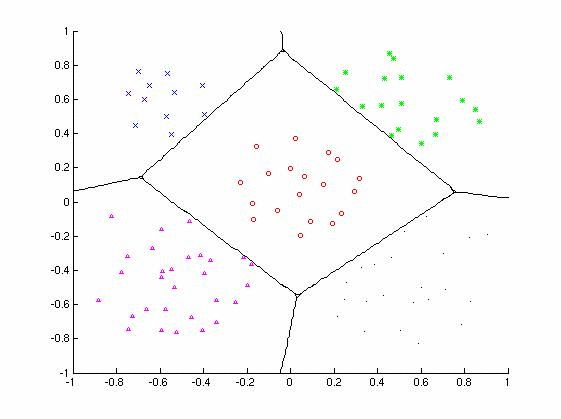### Example: Principal Component Analysis.

 The figure shows the Principal Component Analysis used to find the 1D representation of the input 2D data with the minimal reconstruction error.### Example: Comparison between LDA and PCA.

 The example shows a difference between the Linear Discriminant Analysis and the Principal Component Analysis used for feature extraction.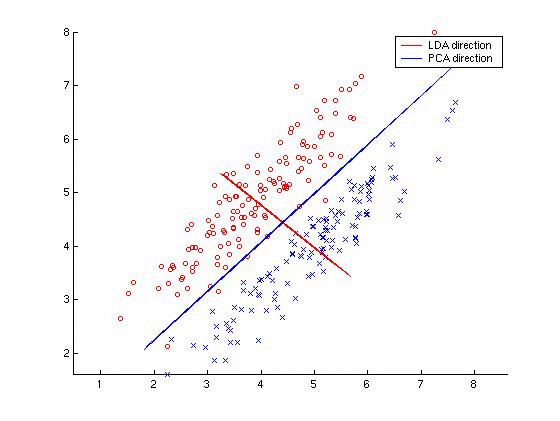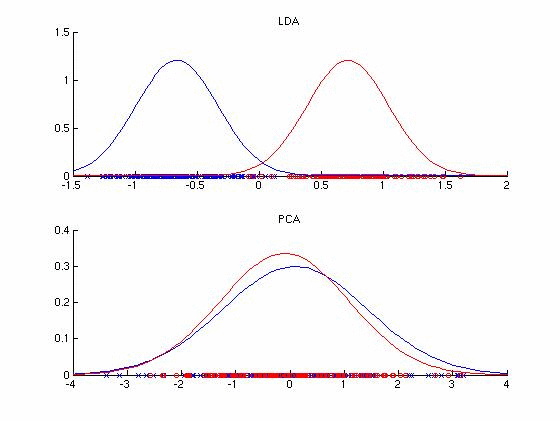### Example: Greedy Kernel Principal Component Analysis.

 The example shows the greedy kernel PCA algorithm used to model the training data.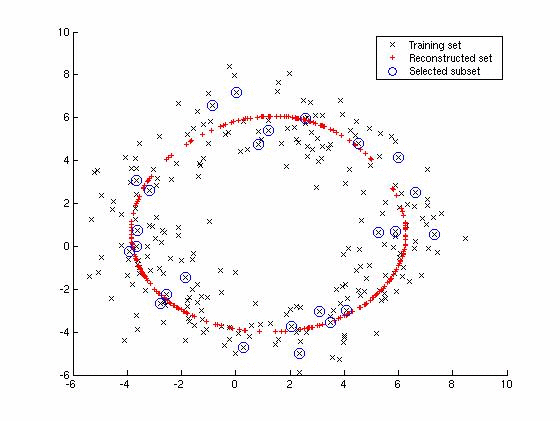### Example: Quadratic classifier trained the Perceptron.

 The figure shows quadratic classifier found by the Perceptron algorithm on the data mapped to the feature by the quadratic mapping.### Example: Probabilistic output for Support Vector Machines.

 The example shows fitting of a posteriori probability to the SVM output. The sigmoid function is fitted by ML estimation and the Gaussian model is used for comparison.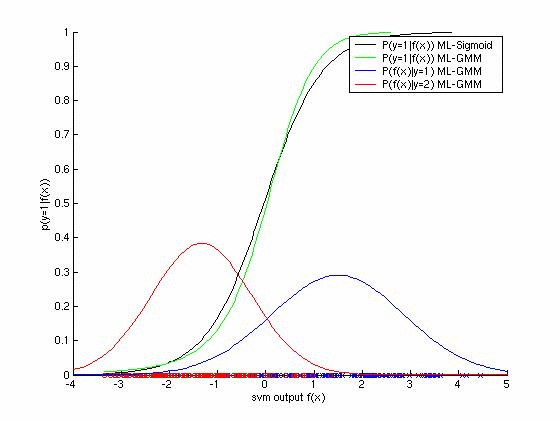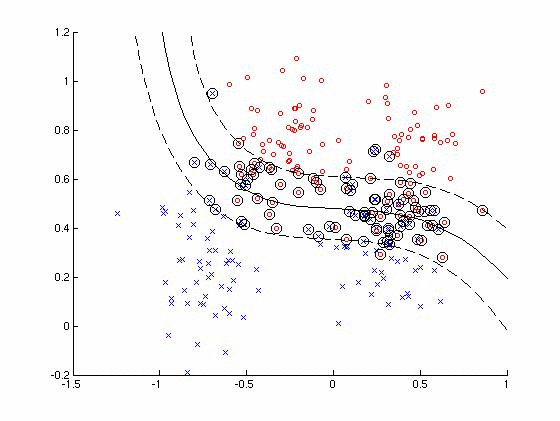### Example: K-means clustering.

 The figure shows data clustering found by the K-means algorithm.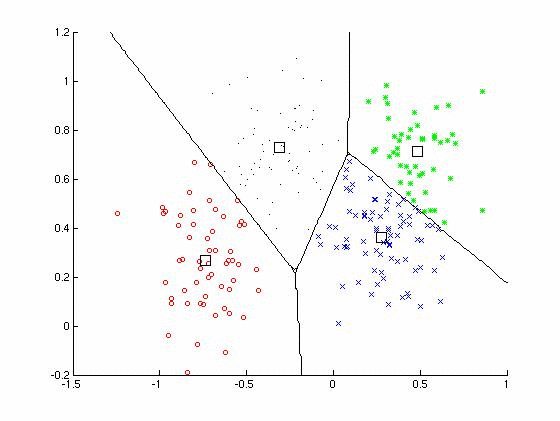### Example: Multi-class BSVM with L2-soft margin.

 The figure showing the multi-class BSVM classifier with L2-soft margin.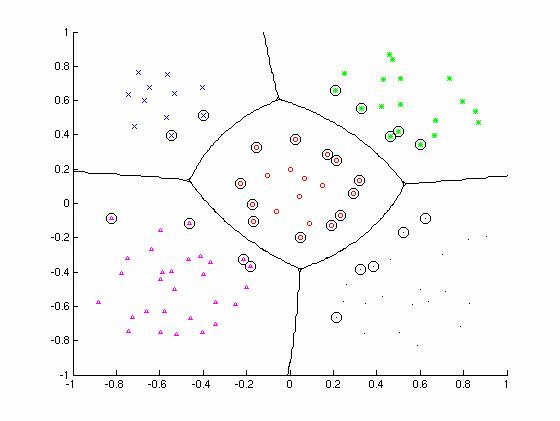### Example: Kernel Fisher Discriminant.

 The figure shows the binary classifier trained based on the Kernel Fisher Discriminant.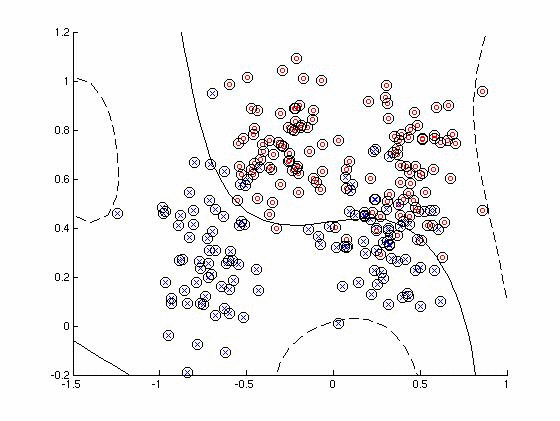### Example: Reduced set method for SVM classifier.

 The figure shows the decision boundary of the SVM classifier and its approximation computed by the reduced set method. The original decision rule involves 94 support vectors while the reduced one only 10 support vectors.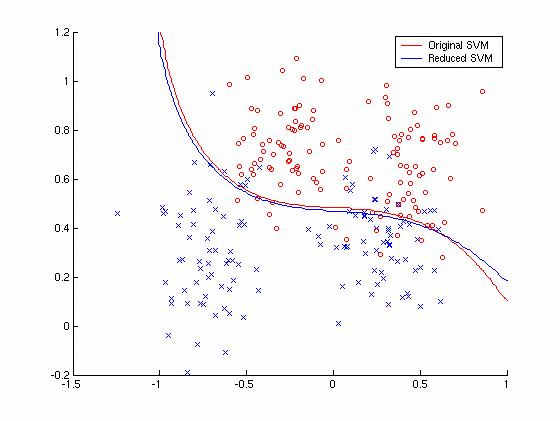### Example: Bayesian classifier with reject option.

 The figure shows the decision boundary of the Bayesian classifier (solid line) and the decision boundary of the reject-option rule with (dashed line). The class-conditional distributions are model by the Gaussian mixture models estimated by the EM algorithm.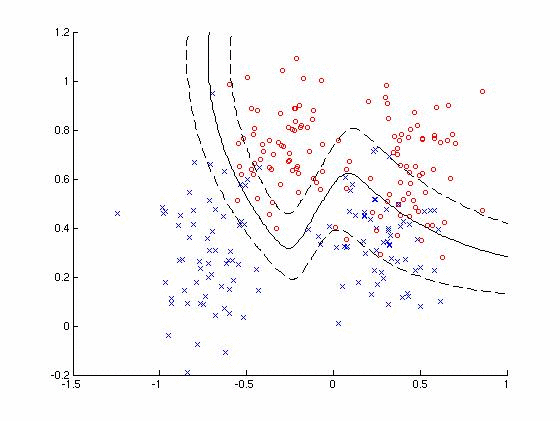### Example: K-nearest neighbors classifier.

 The figure shows the decision boundary of the (K=8)-nearest neighbors classifier.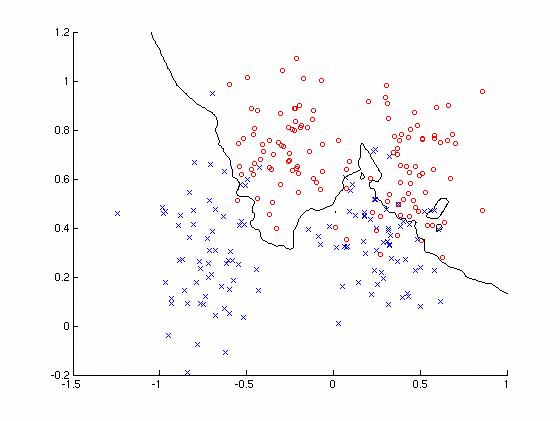### Demo: Optical Character Recognition.

 The toolbox provides means to design the OCR system: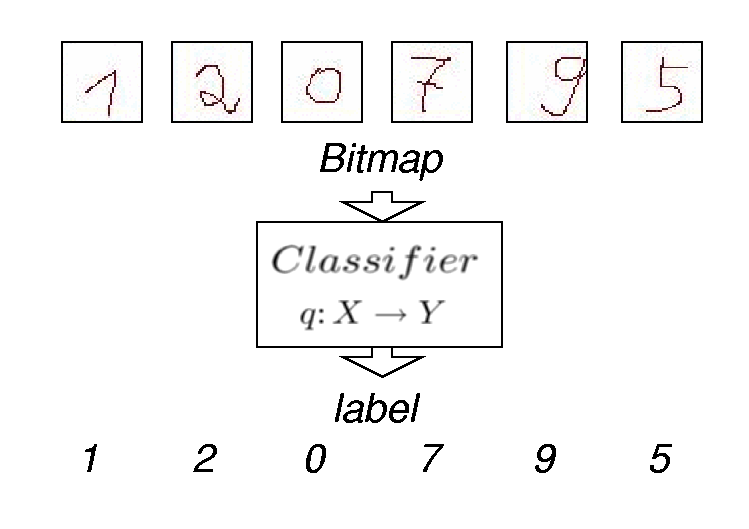The figures show the OCR for the hand-written numerals base on the multi-class SVM. The toolbox provides a simple GUI which allows to draw the numerals by a standard mouse.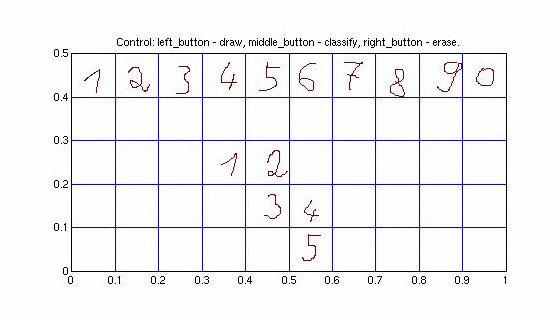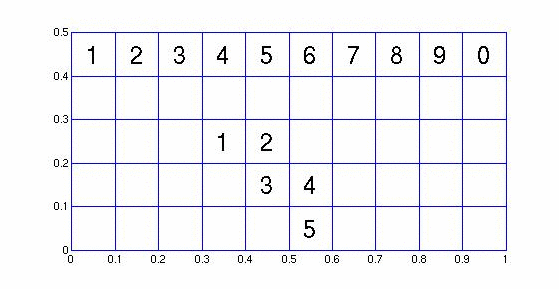### Demo: Image denoising by the kernel PCA.

 The figure shows the idea of using the kernel PCA to model for image denoising.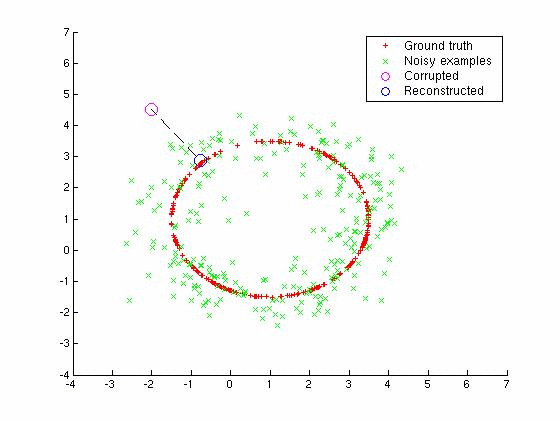The figures shows application of kernel PCA for denoising of the USPS hand-written numerals corrupted by the Gaussian noise.
 Ground truthNoisy images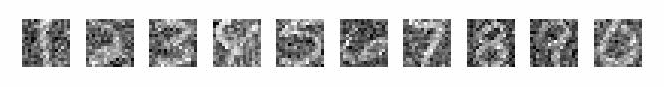Linear PCA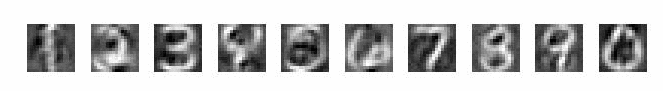Kernel PCA